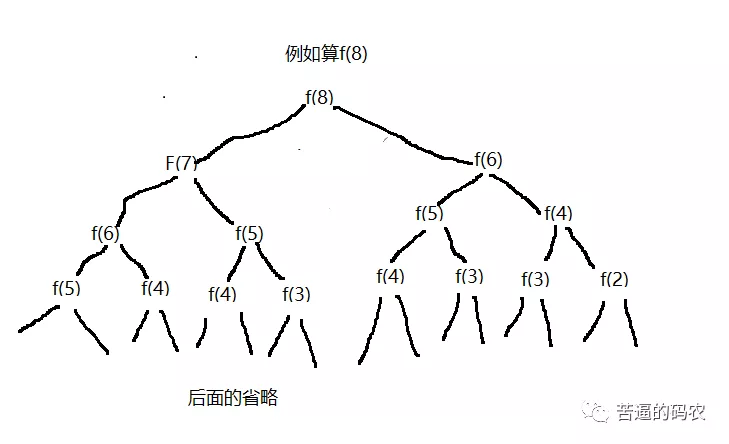# 算法越学越扎心，有没啥破解之法？

### 切勿盲目刷题：刷题前的知识积累

1、常见数据结构：链表、树(如二叉树)。（是的，链表和二叉树是重点，图这些可以先放着）

2、常见算法思想：贪婪法、分治法、穷举法、动态规划，回溯法。（贪婪、穷举、分治是基础，动态规划有难度，可以先放着）

### AC不是目的，我们要追求完美

public int solve(int n){
if(n <= 2){
return n;
}else{
return solve(n-1) + solve(n-2);
}
}所以可以采取下面的方法：

//用一个HashMap来保存已经计算过的状态
static Map<Integer,Integer> map = new HashMap();
public static int solve(int n){
if(n <= 2){
return n;
}else{//是否计算过
if(map.containsKey(n)){
return map.get(n);
}else{
int m = solve(n-1) + solve(n-2);
map.put(n, m);
return m;
}
}
}



public static int solve(int n){
if(n <= 2){
return n;
}
int f1 = 0;
int f2 = 1;
int sum = 0;
for(int i = 1; i<= n; i++){
sum = f1 + f2;
f1 = f2;
f2 = sum;
}
return sum;
}


1、在刷题的时候，我们要力求完美。

2、我想不到这些方法啊，怎么办？那么你就可以去看别人的做法，之后，遇到类似的题，你就会更有思路，更知道往哪个方向想。

3、可以从简单暴力入手做一道题，在考虑空间与时间之间的衡量，一点点去优化。

leetcode有中文版

【算法技巧】位运算装逼指南

### 再说数据结构发重要性

1、链表（如单向链表、双向链表）。

2、树（如二叉树、平衡树、红黑树）。

3、图（如最短路径的几种算法）。

4、队列、栈、矩阵。

### 总结一下吧

1、老铁们，关注我的原创微信公众号「帅地玩编程」，专注于写算法 + 计算机基础知识（计算机网络+ 操作系统+数据库+Linux）。

2、给俺点个赞呗，可以让更多的人看到这篇文章，顺便激励下我，嘻嘻。

©️2019 CSDN 皮肤主题: 程序猿惹谁了 设计师: 上身试试